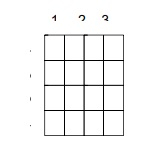# Program to find number of squares in a chessboard in C++

C++Server Side ProgrammingProgramming

#### C in Depth: The Complete C Programming Guide for Beginners

45 Lectures 4.5 hours

#### Practical C++: Learn C++ Basics Step by Step

Most Popular

50 Lectures 4.5 hours

#### Master C and Embedded C Programming- Learn as you go

66 Lectures 5.5 hours

In this problem, we are given the size of a chessboard. Our task is to create a program to find number of squares in a chessboard in C++.

Problem Description − To find the number of squares in a chessboard. We will have to calculate all the combinations of the square that are inside the chessboard i.e. we will consider squares of side 1x1, 2x2, 3x3 … nxn.

Let’s take an example to understand the problem,

Input: n = 4.Output: 30

Squares of size 1x1 -> 16
Squares of size 2x2 -> 9
Squares of size 3x3 -> 4
Squares of size 4x4 -> 1
Total number of squares = 16+9+4+1 = 30

Solution Approach:

A simple approach is by using the sum formula for nxn grid.
Let’s deduct the general formula for the sum,
sum(1) = 1
sum(2) = 1 + 4 = 5
sum(3) = 1 + 4 + 9 = 14
sum(4) = 1 + 4 + 9 + 16 = 30
The sum is can be generalised as
sum = 12 + 22 + 32 + 42 + … n2
sum = ( (n*(n+1)*((2*n) + 1))/6 )

## Example

#include <iostream>
using namespace std;
int calcSquaresCount(int n){
int squareCount = ( (n * (n+1) * (2*n + 1))/6 );
return squareCount;
}
int main() {
int n = 6;
cout<<"The total number of squares of size "<<n<<"X"<<n<<" is
"<<calcSquaresCount(n);
}

## Output:

The total number of squares of size 6X6 is 91